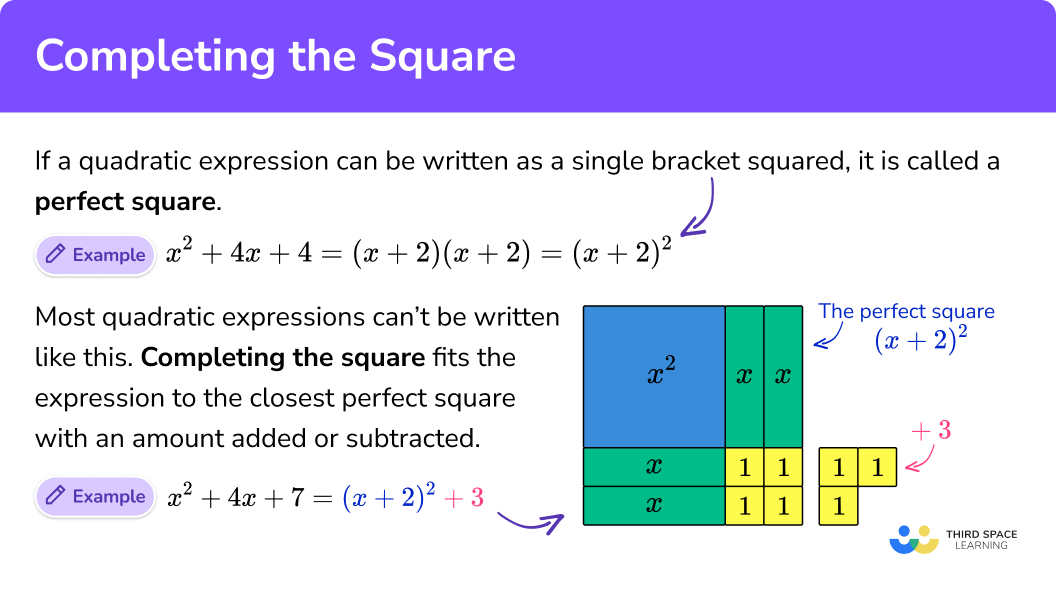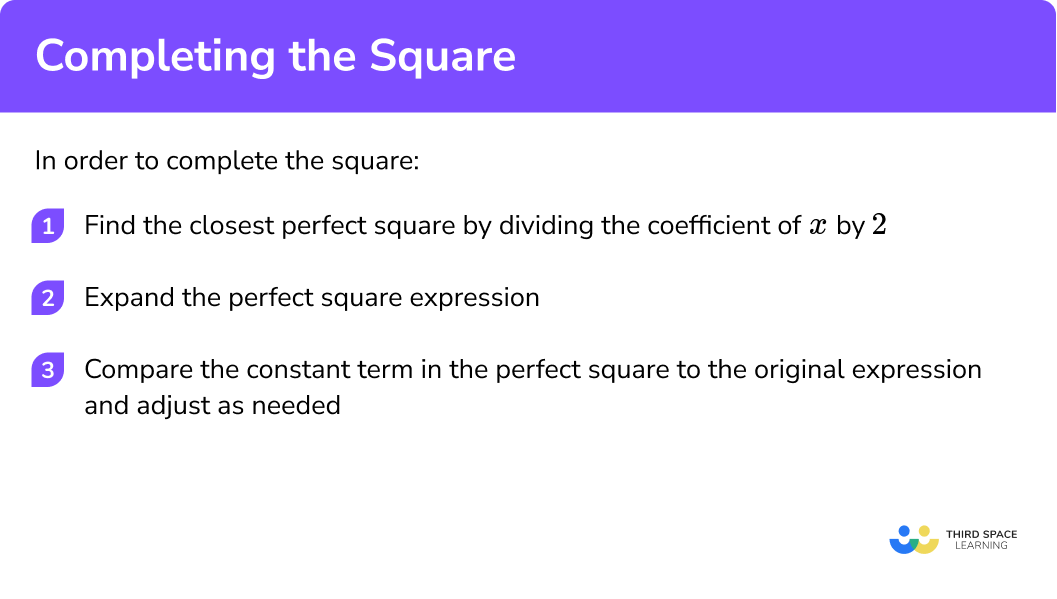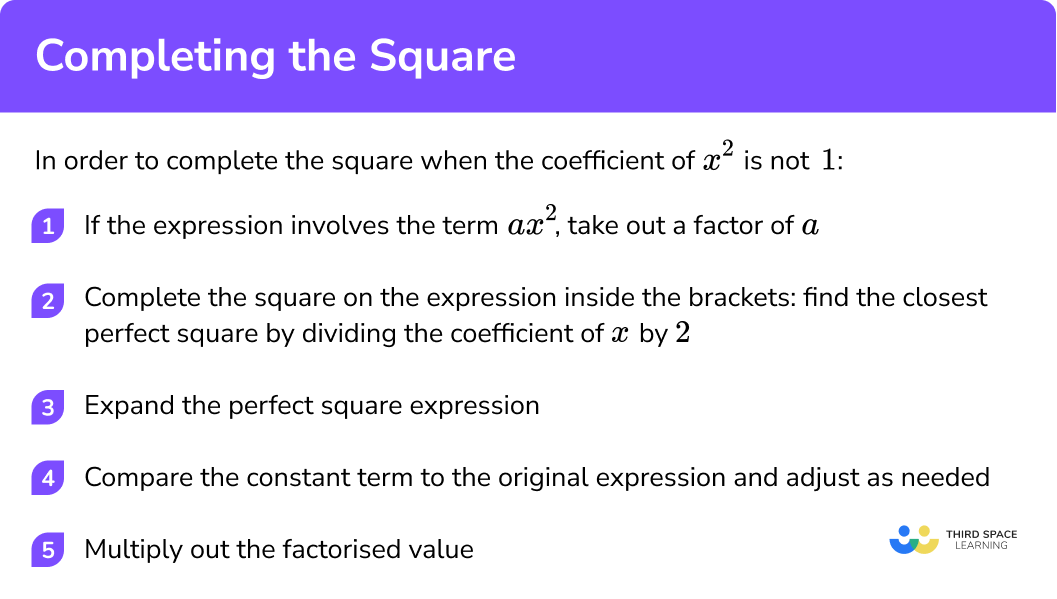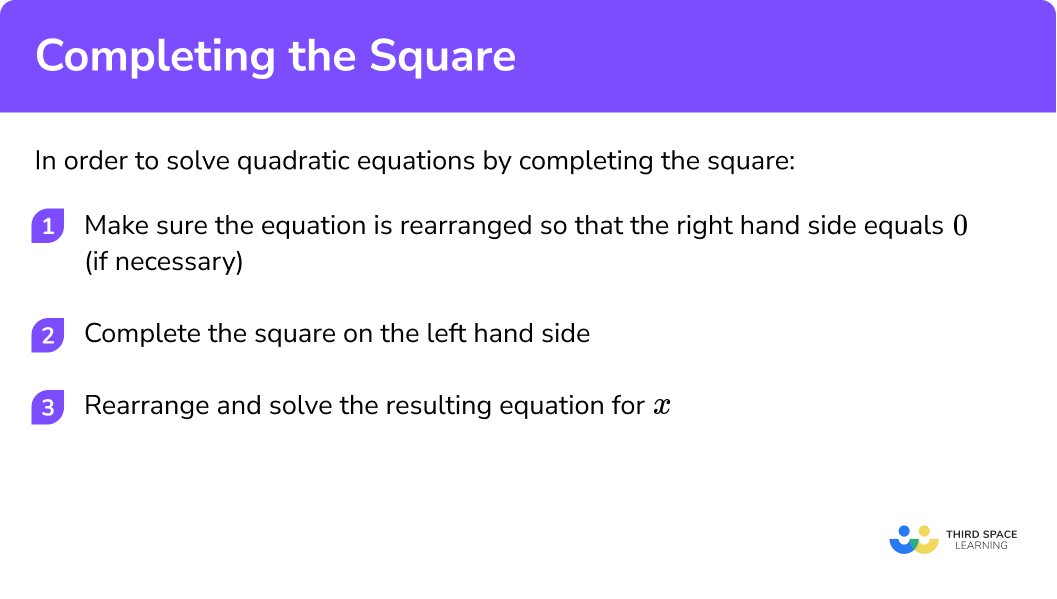# Completing The Square

Here is everything you need to know about completing the square for GCSE maths (Edexcel, AQA and OCR). You’ll learn how to recognise a perfect square, complete the square on algebraic expressions, and tackle more difficult problems with the coefficient of x2 ≠ 1.

You will also learn how to solve quadratic equations by completing the square, and how the completed square form links to graphs of quadratic equations.

Look out for completing the square worksheets and exam questions at the end.

## What is completing the square?

A quadratic expression like x2 + 4x + 4 is called a perfect square.

This is because it factorises to give (x + 2)( x+ 2), which can also be written as (x + 2)2.

We can see this idea diagrammatically as follows:

Most quadratic expressions are not perfect squares, and cannot be written in this form as a single squared bracket. When we complete the square, we try to fit the expression to the closest possible perfect square, with a little bit added or subtracted to make things work.

Some expressions will have an ‘extra’ amount over from a perfect square, such as:

$x^{2} + 4x + 7$

So we would write this expression in completed square form as:

$(x + 2)^{2} + 3$

Some expressions will be ‘missing’ an amount to make a perfect square, such as:

$x^{2} + 6x + 4$

So we would write this expression in completed square form as:

$(x + 3)^{2} – 5$

While it’s easy to see this using diagrams for quadratic expressions with small coefficients, we need a better method for expressions with larger coefficients. You may have noticed already that we divide the coefficient of x by 2 in order to work out the nearest perfect square.

Completing the square is a really useful method for solving quadratic equations; the quadratic formula for solving quadratic equations is based on it and can be derived by completing the square.

The completed square form of a quadratic expression is also really useful for identifying key points of quadratic functions, such as the maximum or minimum of a quadratic parabola (also called the vertex), without having to draw a graph. You can see this in the examples below.

You may sometimes see an expression in the form (x + a) referred to as a binomial.

### What is completing the square?## How to complete the square

In order to complete the square:

1. Find the closest perfect square by dividing the coefficient of x by 2.
2. Expand the perfect square expression.
3. Compare the constant term in the perfect square to the original expression, and adjust as needed.

### Explain how to complete the square in 3 steps### Related lessons on quadratic equations

Completing the square is part of our series of lessons to support revision on quadratic equations and solving equations. You may find it helpful to start with the main solving equations lesson for a summary of what to expect, or use the step by step guides below for further detail on individual topics. Other lessons in this series include:

## Completing the square examples

### Example 1: perfect square

Complete the square for the expression

$x^{2} + 8x + 16$

1. Find the closest perfect square by dividing the coefficient of x by 2.

The coefficient of x is 8, so when we divide this by 2, we get 4.

The closest perfect square is:

$(x + 4)^{2}$

2 Expand the perfect square expression.

$(x + 4)^{2} = x^{2} + 8x + 16$

3 Compare the constant term in the perfect square to the original expression, and adjust as needed.

These match (because our example was a perfect square), so we don’t need to make any adjustment.

The answer in complete square form is

$(x + 4)^{2}$

Graphically

This graph shows the curve

$y = x^{2} + 8x+ 16$

The minimum value of y occurs when the bracket equals 0. This happens when x = −4.

If we substitute x = −4, we get:

$y = (-4 + 4)^{2} = 0^{2} = 0$

So the coordinates of the vertex, which is a minimum point, are (−4, 0).

### Example 2: simple case with coefficient of x2 = 1 and an even, positive coefficient of x

Complete the square for the expression

$x^{2} + 6x + 17$

The coefficient of x is 6, so when we divide this by 2, we get 3.

The closest perfect square is

$(x + 3)^{2}$

$(x + 3)^{2} = x^{2} + 6x + 9$

In order to make the constant term correct, we need to add 8, because 9 + 8 = 17.

The answer in complete square form is

$(x + 3)^{2} + 8$

Graphically

This graph shows the curve

$y = x^{2} + 6x + 17$

The minimum value of y occurs when the bracket equals 0. This happens when x = −3.

If we substitute x = −3, we get:

$y = (-3 + 3)^{2} + 8 = 0^{2} + 8 = 8$

So the coordinates of the vertex, which is a minimum point, are (−3, 8).

As a little shortcut, the y value is just whatever number is on its own outside the bracket, and the x value is the opposite sign of the number inside the bracket.

### Example 3: another simple case with coefficient of x2 = 1 and an even, positive coefficient of x

Complete the square for the expression

$x^{2} + 2x – 5$

The coefficient of x is 2, so when we divide this by 2, we get 1.

The closest perfect square is

$(x +1)^{2}$

$(x + 1)^{2} = x^{2} + 2x + 1$

In order to make the constant term correct, we need to subtract 6, because 1 − 6 = −15.

The answer in complete square form is

$(x + 1)^{2} – 6$

Graphically

This graph shows the curve

$y = x^{2} + 2x – 5$

The minimum value of y occurs when the bracket equals 0. This happens when x = −1.

If we substitute x = −1, we get:

$y = (-1 + 1)^{2} – 6 = 0^{2} – 6 = -6$

So the coordinates of the vertex, which is a minimum point, are (−1, −6).

### Example 4: coefficient of x2 = 1  and a negative coefficient of x

This is really straightforward – just remember that your perfect square bracket will need subtraction rather than addition in the middle.

Complete the square for the expression

$x^{2} – 10x + 17$

The coefficient of x is −10, so when we divide this by 2, we get −5.

The closest perfect square is

$(x – 5)^{2}$

$(x – 5)^{2} = x^{2} – 10x + 25$

In order to make the constant term correct, we need to subtract 8, because 25 − 8 = 17.

The answer in complete square form is

$(x – 5)^{2} – 8$

Graphically

This graph shows the curve

$y = x^{2} – 10x + 17$

The minimum value of y occurs when the bracket equals 0. This happens when x = −5.

If we substitute x = 5, we get:

$y = (5 – 5)^{2} – 8 = 0^{2} – 8 = -8$

So the coordinates of the vertex, which is a minimum point, are (5, −8).

### Example 5: coefficient of x2 = 1 and an odd coefficient of x

Complete the square for the expression

$x^{2} + 3x + 4$

The coefficient of x is 3, so when we divide this by 2, we get

$\frac{3}{2}$

It can be tempting to use decimals, but fractions are much easier, particularly as completing the square is more likely to be examined on a non-calculator paper at GCSE.

The closest perfect square is

$\left(x + \frac{3}{2}\right)^{2}$

$\left(x + \frac{3}{2}\right)^{2} = x^{2} + 3 x + \frac{9}{4}$

It can be useful to think of 4 as the improper fraction

$\frac{16}{4}$

this makes it easier to work out the adjustment.

In order to make the constant term correct, we need to add

$\frac{7}{4}$

because

$\frac{9}{4} + \frac{7}{4} = \frac{16}{4} = 4$

The answer in complete square form is

$\left(x+\frac{3}{2}\right)^{2}+\frac{7}{4}$

Graphically

This graph shows the curve

$y=x^{2}+3x+4$

The minimum value of y occurs when the bracket equals 0. This happens when

$x=\frac{-3}{2}$

If we substitute

$x=\frac{-3}{2}$

we get:

$y=\left(\frac{-3}{2}+\frac{3}{2}\right)^{2}+\frac{7}{4}=0^{2}+\frac{7}{4}=\frac{7}{4}$

So the coordinates of the vertex, which is a minimum point, are

$\left(\frac{-3}{2}, \frac{7}{4}\right)$

## How to complete the square when coefficient of x2 is not 1²

If the coefficient of x2 is not 1, we must first factorise to get an expression where the coefficient of x2 is 1. If the expression involves the term ax2, then we take out a factor of a.

1. Factorise.
2. Complete the square on the expression inside the brackets: find the closest perfect square by dividing the coefficient of x by 2.
3. Expand the perfect square expression.
4. Compare the constant term in the perfect square to the original expression, and adjust as needed.
5. Multiply out the factorised value.

### How to complete the square when coefficient of x² is not 1## Completing the square when coefficient not 1 example

### Example 6: coefficient of x2 ≠ 1

Complete the square for the expression

$2x^{2} + 8x – 10$

We take out the common factor of 2, so the expression becomes

$2\left(x^{2} + 4 x-5\right)$

then complete the square on the expression inside the bracket.

The coefficient of x is 4, so when we divide this by 2, we get 2.

The closest perfect square is

$(x+2)^{2}$

$(x+2)^{2}=x^{2}+4x+4$

In order to make the constant term correct, we need to subtract 9, because 4 − 9 = −5.

So the expression in complete square form is

$(x+2)^{2}-9$

Don’t forget to deal with the factor of 2 that we removed at the start. So the expression:

$2\left(x^{2}+4 x-5\right)$

in complete square form is:

$2\left((x+2)^{2}-9\right)$

Finally, expand out to give a final answer:

$2(x+2)^{2}-18$

Graphically

This graph shows the curve

$y=2x^{2}+8x-10$

The minimum value of y occurs when the bracket equals 0. This happens when x = −2.

If we substitute x = −2, we get:

$y=2(x+2)^{2}-18=0^{2}-18=-18$

So the coordinates of the vertex, which is a minimum point, are (−2, −18).

Note: we can also complete the square for expressions with a negative x2 coefficient in the same way, by taking out a negative factor in the first step.

For example,

$-x^{2}+5x-3$

would be written as:

$-\left(x^{2}-5 x+3\right)$

and then completed as in Example 5.

## How to solve quadratic equations using completing the square

Some quadratic equations can be solved by factorising, but most require either completing the square or the quadratic formula – in fact, the quadratic formula is derived from completing the square (see worksheet).

In order to solve quadratic equations using complete the square:

1. Make sure the equation is rearranged so that the right hand side equals 0 (if necessary).
2. Complete the square on the left hand side.
3. Rearrange and solve the resulting equation for x.

### How to solve quadratic equations using completing the square## Solving using completing the square method examples

### Example 7: solving a quadratic by completing the square

$x^{2}+6x+3=0$

RHS already equals 0 here, so no need to do anything.

$x^{2}+6x+3=(x+3)^{2}-6$

\begin{aligned} (x+3)^{2}-6&=0 \\\\ (x+3)^{2}&=6 \\\\ x+3&=\pm \sqrt{6} \\\\ x&=-3 \pm \sqrt{6} \end{aligned}

We have two solutions to the equation:

$x=-3+\sqrt{6} \\\\ x=-3-\sqrt{6}$

It is really important to remember the ± sign when square-rooting, as most quadratic equations you’re asked to solve at GCSE have two solutions or roots. Sometimes, there will be one repeated root.

### Example 8: solving a harder quadratic by completing the square

$2x^{2}+10x=-5-2x$

Rearrange by adding 5 and 2x to both sides of the equation to give

$2x^{2}+12x+5=0$

Remember to take out a common factor of 2 first.

\begin{aligned} 2 x^{2}+12 x+5 &=2\left[x^{2}+6 x+\frac{5}{2}\right] \\\\ &=2\left[(x+3)^{2}-\frac{13}{2}\right] \\\\ &=2(x+3)^{2}-13 \end{aligned}

\begin{aligned} 2(x+3)^{2}-13&=0 \\\\ 2(x+3)^{2}&=13 \\\\ (x+3)^{2}&=\frac{13}{2} \\\\ x+3&=\pm \sqrt{\frac{13}{2}} \\\\ x&=-3 \pm \sqrt{\frac{13}{2}} \end{aligned}

We have two solutions to the equation:

$x=-3+\sqrt{\frac{13}{2}} \\\\ x=-3-\sqrt{\frac{13}{2}}$

### Common misconceptions

The sign will always be the same sign as the coefficient of x.

• Errors with fraction arithmetic

In particular, remember to square the numerator and denominator when squaring a fraction.

• Going the wrong way when working out the adjustment

Remember to always work out how you get from the perfect square to the expression in the question.

• Forgetting the ± sign when using the completing the square method to solve quadratics

Check carefully to make sure you haven’t lost one of your solutions!

### Practice completing the square questions

1. Complete the square:

x^{2} + 12x + 36

(x+12)^{2}(x+6)^{2}(x-6)^{2}(x+6)^{2} +36Half the coefficient of x is 6 so it is (x+6)^{2} .

Expanding (x+6)^{2}. gives us x^{2}+12x+36 so we do not need to add or subtract anything.

2. Complete the square:

x^{2}+6x+2

(x+3)^{2}-7(x+3)^{2}+7(x-3)^{2}-7(x+1)^{2}+1Half the coefficient of x is 3 so it is (x+3)^{2} .

Expanding (x+3)^{2} gives us x^{2}+6x+9 so we need to subtract 7 which gives us (x+3)^{2} -7 .

3. Complete the square:

x^{2}-5x+12

=(x-5)^{2}-13=(x+\frac{5}{2})^{2}+\frac{23}{4}=(x-\frac{5}{2})^{2}+\frac{73}{4}=(x-\frac{5}{2})^{2}+\frac{23}{4}Half the coefficient of x is \frac{5}{2} so it is (x-\frac{5}{2})^{2} .

Expanding (x-\frac{5}{2})^{2} gives us x^{2}-5x+\frac{25}{4} .

We need +12 , which is the same as + \frac{48}{4} so we need to add \frac{23}{4} .

Therefore the answer is (x-\frac{5}{2})^{2} + \frac{23}{4} .

4. Complete the square:

-2x^{2}-4x+14

2(-x-2)^{2}+20-2(x+1)^{2}+16-(2x-2)^{2}+10-2(x-1)^{2}-26We must first take a factor of -2 out to get -2(x^{2}+2x-7) .

We then need to complete the square for x^{2}+2x-7 .

This gives us (x+1)^{2}-8 .

So the final answer is -2[(x+1)^{2}-8]=-2(x+1)^{2}+16 .

5. Find the coordinates of the minimum point of the function:

y=x^{2}-6x+1

(6, -35)(-3, -8)(3, -8)(-6, 1)We need to start by completing the square. This gives us y=(x-3)^{2}-8 .

We can then see that the minimum point is when   (x-3) = 0 so x=3 .

Substituting this in gives us y=0^{2}-8=-8. .

Therefore the minimum point is (3, -8) .

6. By first completing the square, find the solutions to the equation:

x^{2}+8x+3=0

x=-4 \pm \sqrt{13}x=-4 \pm \sqrt{3}x=4 \pm \sqrt{13}x=-4 + \sqrt{13}Completing the square gives us (x+4)^{2}-13 .

Therefore we need to solve:

\begin{aligned} (x+4)^{2}-13&=0\\\\ (x+4)^{2}&=13\\\\ x+4&=\pm \sqrt{13}\\\\ x&= -4 \pm \sqrt{13} \end{aligned}

### Completing the square GCSE questions

1.  x^{2}+12x+19   can be written in the form

(x+a)^{2}+b   where  a   and  b   are integers.

Work out the values of  a   and  b .

(2 marks)

a = 6

(1)

b = −17

(1)

2.  (a)    x^{2}-6x+7 can be written in the form

(x+a)^{2}+b   where  a   and  b   are integers.

Work out the values of  a   and  b .

(b)   Hence, or otherwise, solve the equation

x^{2}-6x+7=0

(4 marks)

(a)

a = −3

(1)

b = −2

(1)

(b)

x = 3 + \sqrt{2}

(1)

x = 3  −  \sqrt{2}

(1)

3.  (a)  3x^{2}+12x-18   can be written in the form

a(x+b)^{2}+c   where  a   and  b   are integers.

Work out the values of  a, b   and  c .

(b)   Hence, or otherwise, find the coordinates of the turning point of the graph of  y=3x^{2}+12x-18

(4 marks)

(a)

a = 3

(1)

b = 2

(1)

c = −30

(1)

(b)

(−2, −30)

(1)

## Learning checklist

You have now learned how to:

• Complete the square for a quadratic expression
• Solve quadratic equations by completing the square
• Identify turning points by completing the square

## Still stuck?

Prepare your KS4 students for maths GCSEs success with Third Space Learning. Weekly online one to one GCSE maths revision lessons delivered by expert maths tutors.

Find out more about our GCSE maths tuition programme.